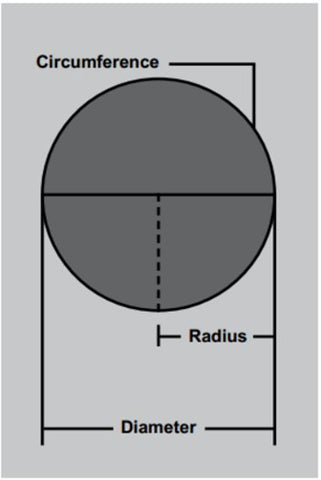507-218-8976 | info@valleysupplymn.com

# Helpful FormulasArea of a Square/Retangle

Length x Width = sq./ft.

Circle Circumference

3.14 x Diameter = Circle Circumference

3.14 x Radius x 2 = Circle Circumference

Area of a Circle

Radius x Radius x 3.14 = Area of a Cirlce